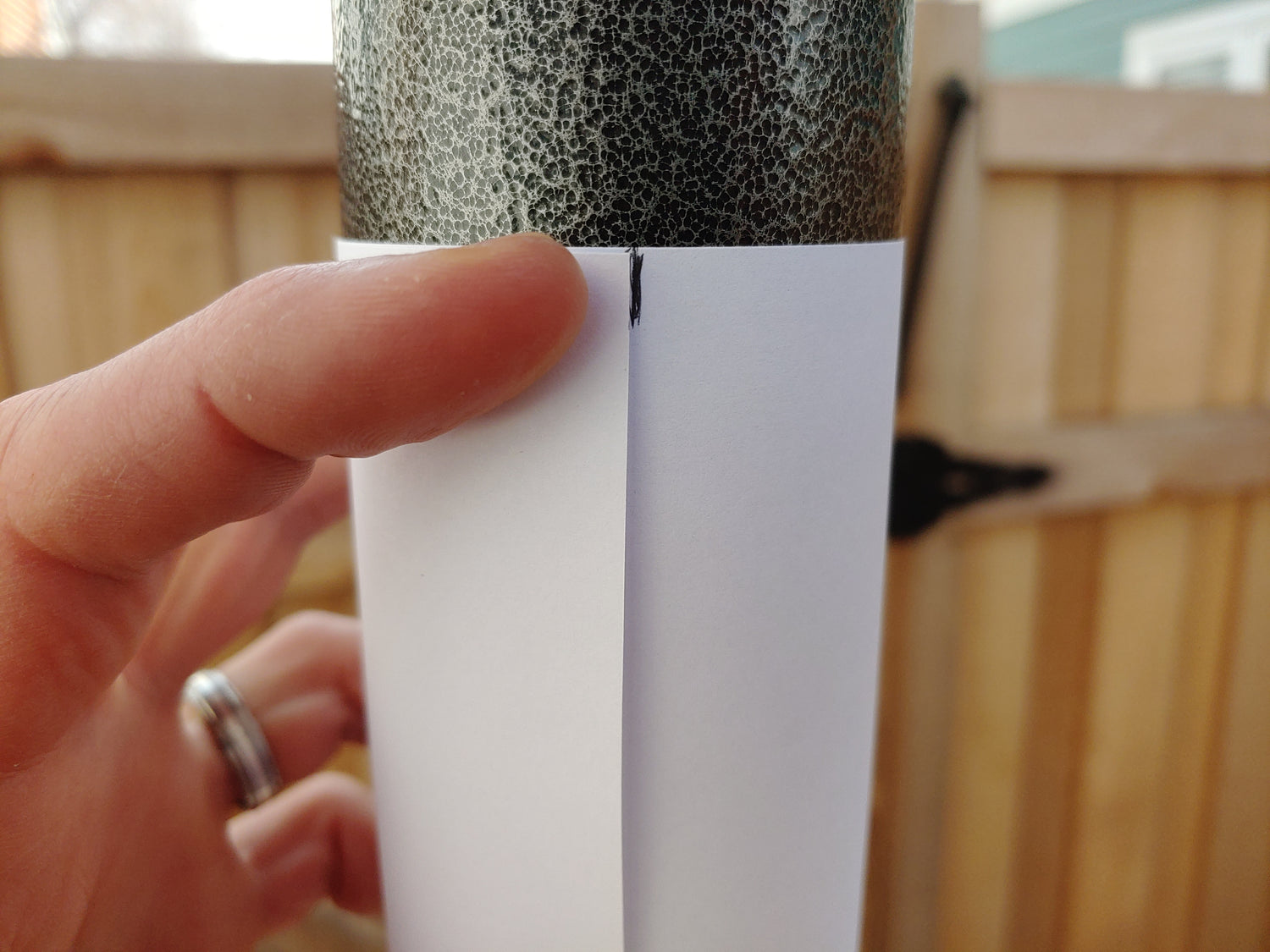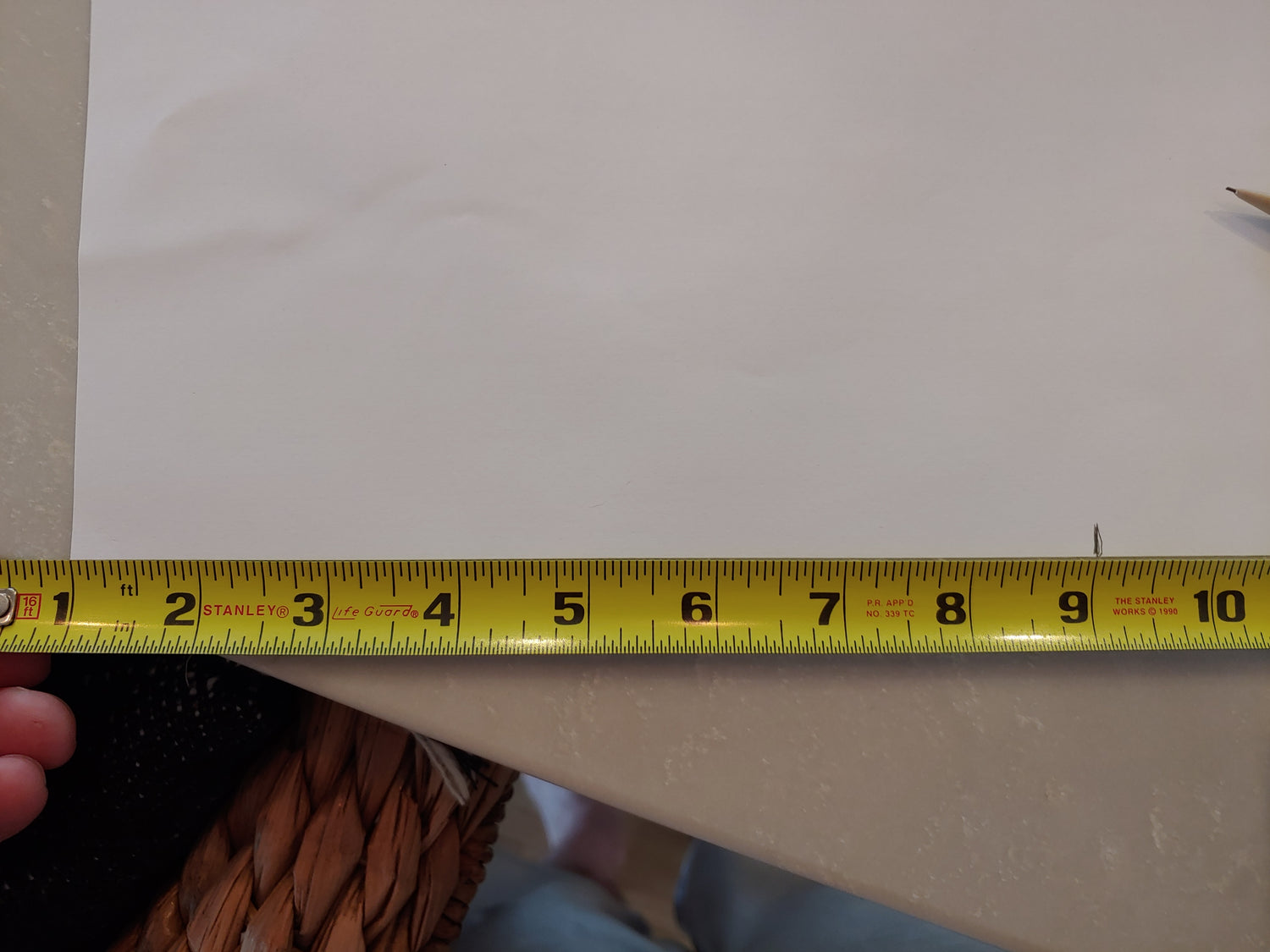## 1) Wrap and mark

Tightly wrap a piece of paper around the pole and mark where one side starts to overlap.## 2) Measure

Open the paper and measure the distance as accurately as possible (to the 1/16"or 1/32").

Tip - rather than measuring from the end of the tape measure which might not be straight, measure from the "1". Don't forget to subtract 1 from the next step :)## 3) Calculate

This measurement will equal the circumference. Divide the measurement by 3.14 to determine the diameter.

In this example, the circumference is 8 1/8 (after subtracting "1"), so the diameter is 2.58". This will fit the 2.5" pole flange.